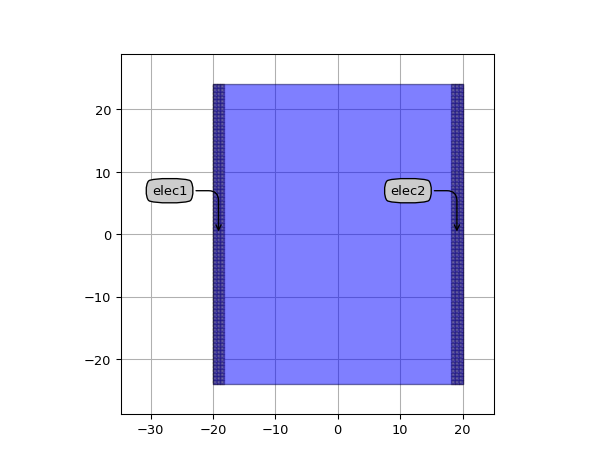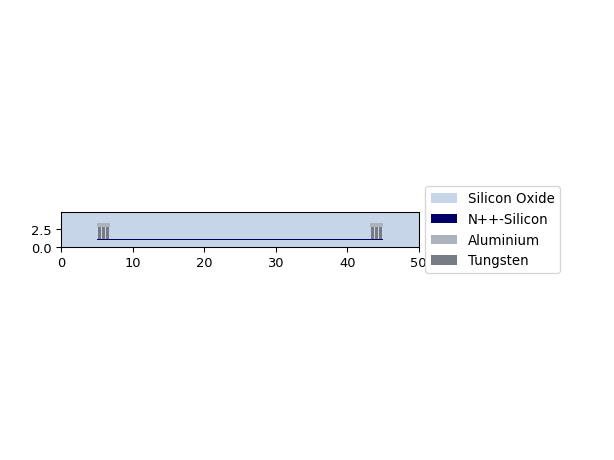# Resistor¶

SiFab contains one resistor.

## Resistor¶

This resistor is built on the NPLUS doped silicon layer. It has the following properties:

• resistance
• length
• contact_pitch
• n_contact_rows

The width of the resistor is calculated based on the sheet resistance of the NPLUS silicon layer, estimated at 60 $$\Omega / \square$$.

from si_fab import all as pdk
from ipkiss3 import all as i3

# Resistor
r = pdk.Resistor(
resistance=50.0,
length=40.0,
n_contact_rows=3,
contact_pitch=0.6,
)
r_lv = r.Layout()
r_lv.visualize(annotate=True)

xs = r_lv.cross_section(cross_section_path=i3.Shape([(-25.0, 1.0), (25, 1.0)]))
xs.visualize()